Topics: AI | Data Science | Data Visualization | Deep Learning | Machine Learning | NLP | Python | R | Statistics

KDnuggets Home » News » 2021 » Aug » Tutorials, Overviews » Linear Algebra for Natural Language Processing ( 21:n31 )

# Linear Algebra for Natural Language Processing

Learn about representing word semantics in vector space.

By Taaniya Arora, Data ScientistPhoto by Michael Dziedzic on Unsplash

The field of Natural Language Processing involves building techniques to process text in natural language by people like you and me, and extract insights from it for performing a variety of tasks from interpreting user queries on search engines and returning web pages, to solving customer queries as chatbot assistant. The importance of representing every word into a form that captures the meaning of the word and the overall context becomes crucial especially when major decisions are based upon the insights extracted from text on a large scale — like forecasting stock price change with social media.

In this article, we’ll begin with the basics of linear algebra to get an intuition of sof vectors and their significance for representing specific types of information, the different ways of representing text in vector space, and how the concept has evolved to the state of the art models we have now.

We’ll step through the following areas -

• Unit vectors in our coordinate system
• Linear combination of vectors
• Span in vector coordinate system
• Collinearity & multicollinearity
• Linear dependence and independence of vectors
• Basis vectors
• Vector Space Model for NLP
• Dense Vectors

## Unit vectors in our coordinate system

i-> Denotes a unit vector (vector of length 1 unit) pointing in the x-direction

j -> Denotes a unit vector in the y-direction

Together, they are called the basis of our coordinate vector space.

We’ll come to the term basis more in the subsequent parts below.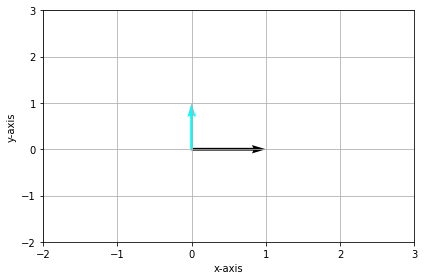Standard Unit vectors — Image by Author

• Suppose we have a vector 3i+ 5j
• This vector has x,y coordinates : 3 & 5 respectively
• These coordinates are the scalars that flip and scale the unit vectors by 3 & 5 units in the x & y directions respectively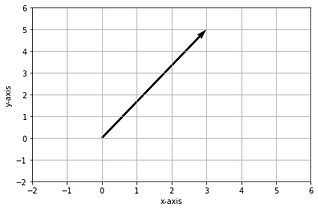A vector in 2D X-Y space — Image by Author

## Linear Combination of 2 vectors

If u & v are two vectors in a 2 dimensional space,then their linear combination resulting into a vector l is represented by -

l = x1. u + x2. v

• The numbers x1x2 are the components of a vector x
• This is essentially a scaling and addition operation by x on the given vectors.

The above expression of linear combination is equivalent to the following linear system -

Bx = l

Where B denotes a matrix whose columns are u and v.

Let’s understand this by an example below with vectors u & v in a 2 dimensional space -

``````# Vectors u & v
# The vectors are 3D, we'll only use 2 dimensions
u_vec = np.array([1, -1, 0])
v_vec = np.array([0, 1, -1])# Vector x
x_vec = np.array([1.5, 2])# Plotting them
# fetch coords from first 2 dimensions
data = np.vstack((u_vec, v_vec))[:,:2]
origin = np.array([[0, 0, 0], [0, 0, 0]])[:,:2]
plt.ylim(-2,3)
plt.xlim(-2,3)
QV = plt.quiver(origin[:,0],origin[:,1], data[:, 0], data[:, 1], color=['black', 'green'], angles='xy', scale_units='xy', scale=1.)
plt.grid()
plt.xlabel("x-axis")
plt.ylabel("y-axis")
plt.show()``````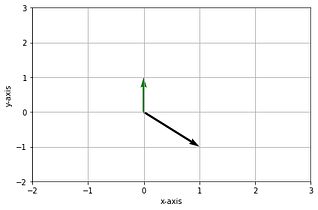Linear Combination of vectors — Image by Author

We can also understand it from this explanation for a similar example with 3 dimensions given by a Professor in his notes here -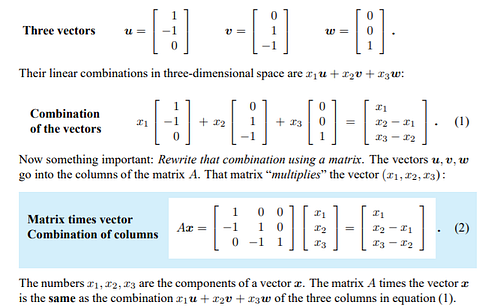Linear Algebra, Chapter 1- Introduction to Vectors, MIT

Taking the 3 vectors from the example in the image and plotting them in 3D space (The units of axes are different than the vectors in the plot)

``````u_vec = np.array([1, -1, 0])
v_vec = np.array([0, 1, -1])
w_vec = np.array([0, 0, 1])data = np.vstack((u_vec, v_vec, w_vec))
origin = np.array([[0, 0, 0], [0, 0, 0], [0, 0, 0]])
fig = plt.figure()
ax.quiver(origin[:,0],origin[:,1], origin[:,2], data[:, 0], data[:, 1], data[:,2])ax.set_xlim([-1, 2])
ax.set_ylim([-1, 2])
ax.set_zlim([-1, 2])
plt.grid()
plt.show()``````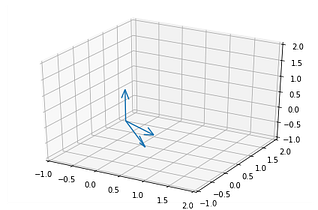Vectors in 3D space — Image by Author

## Span

• A span is a set of all possible combinations of vectors that we can reach with a linear combination of a given pair of vectors
• The span of most pairs of 2-D vectors is all vectors in the 2-D space. Except, when they line up in the same direction (i.e if they are collinear) , in which case, their span is a line.

i.e span( ab) = R² (all vectors in 2D space) , provided they are not collinear.

## Collinearity

Collinearity is the case when we have p different predictor variables but some of them are linear combinations of others, so they don’t add any other information. 2 collinear vectors / variables will have correlation close to +/- 1 and can be detected by their correlation matrix.

Multicollinearity exists when more than 2 vectors are collinear and any pair of vectors may not necessarily have high correlation.

Linear Independence

We say that v1 , v2, . . . , vn are linearly independent, if none of them is

a linear combination of the others. This is equivalent to saying

that x1.v1 + x2.v2 + . . . + xn.vn = 0 implies x1 = x2 = . . . = xn = 0

Since collinear vectors can be expressed as linear combinations of each other, they are linearly dependent.

## Basis

A basis is that set of linearly independent vectors that span that space.

We call these vectors as basis vectors

## Vector space Models in NLP

Vector space is a set V of vectors, where two operations — vector addition and scalar multiplication are defined. For E.g. IF two vectors u & v are in space V, then their sum, w = u + v will also lie in the vector space V.

A 2D vector space is a set of linearly independent vasis vectors with 2 axes.

Each axis represents a dimension in the vector space.

Recalling the previous plot of vector a = (3,5) = 3 i + 5 j again. This vector is represented on a 2D space with 2 linearly independent basis vectors — X & Y, who also represent the 2 axes as well as the 2 dimenions of the space.

3 & 5 here are the x,y components of this vector for representation on the X-Y 2D space.Vector in 2D X-Y plane — Image by Author

## Vector space model in NLP

A vector space model is a representation of text in vector space.

Here, each word in a corpus is a linearly independent basis vector and each basis vector represents an axis in the vector space.

• This means, each word is orthogonal to other words/axes.
• For a corpus of vocabulary |V|will contain |V| axes.
• Combination of terms represent documents as points or vectors in this space

For 3 words, we’ll have a 3D vector model represented like this -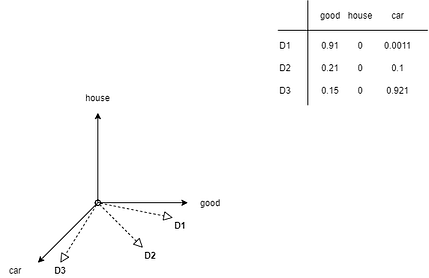Vector Space Model for 3 words — Image by Author

The table above the graph represents the TF-IDF incident matrix.

D1 = (0.91, 0, 0.0011) represents a document vector in the 3 axes — good, house, car. Similarly, we have D2 & D3 document vectors.

How does representation in vector space help us, though?

• One of the common application using this representation is information retrieval for search engines, question answering systems and much more.
• By representing the text into vectors, we aim to use vector algebra to extract semantics from the text and use it for different applications like searching documents containing the similar sementics as those contained in a given search query.

For eg. For a search token ‘buy’ , we would want to get all the documents containing different forms of this word — buying, bought and even synonyms of the word ‘buy’. Such documents can not be captured from other rudimentary methods representing documents as Binary incident matrix.

This is achieved through distance metrics like cosine similarity between vectors of document & query, where the documents closer to the query are ranked the highest.

• The number of words / vocabulary size can be as huge as in millions eg. Google news corpus is 3 Million, which means as many independent axes/dimensions to represent the vectors. Hence, we want to use the operations in vector space to reduce the number of dimensions and bring words with similar to each other in the same axis.

## Dense vectors

• The above operations are possible on document vectors which are represented by extending the above vector representation of documents to documents represented as distributed or dense vectors. These representations capture the semantics of the text and also captures the linear combinations of word vectors
• The previous vector space model with each word representing a separate dimension results in sparse vectors.
• Dense vectors captures the context in the vector representation. The dense vector of words are such that words appearing in similar contexts will have similar representations.
• These dense vectors are also called as word embeddings or distributed representations.
• word2vec is one such framework to learn dense vectors of words from large corpus. It has 2 variants — skip-gram and CBOW(continuous bag of words)
• Some of the other frameworks and techniques to obtain dense vectors are Global Vectors(GloVe), fastText, ELMo(Embeddings from Language Model) and most recent state of the art Bert based approached to obtain contextualized word embeddings during inference.

## Conclusion

This article introduced the concept of vector space based on linear algebra and highlighted the related concepts as part of their application in Natural Language Processing for representing text documents in semantics representation and extraction tasks.

The applications of word embeddings have been extended to more advanced and wide applications with much improved performance than earlier.

You can also refer to the source code and run on colab by accessing my Git repository here.

Hope you enjoyed reading it. :)

## References

Bio: Taaniya Arora is a Data Scientist and problem solver, especially interested in NLP and reinforcement learning.

Original. Reposted with permission.

Related:

Top Stories Past 30 Days
Most Popular
Most SharedGet KDnuggets, a leading newsletter on AI, Data Science, and Machine Learning